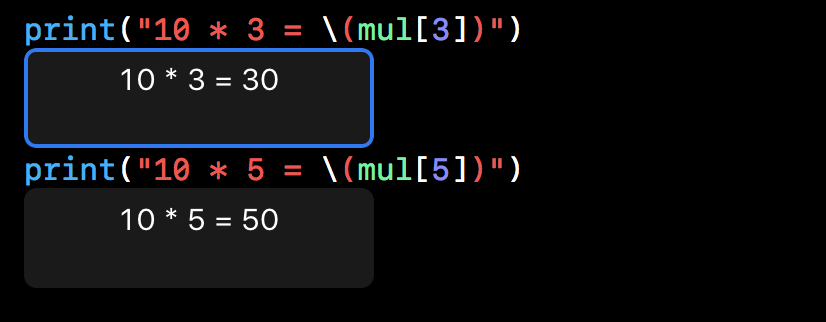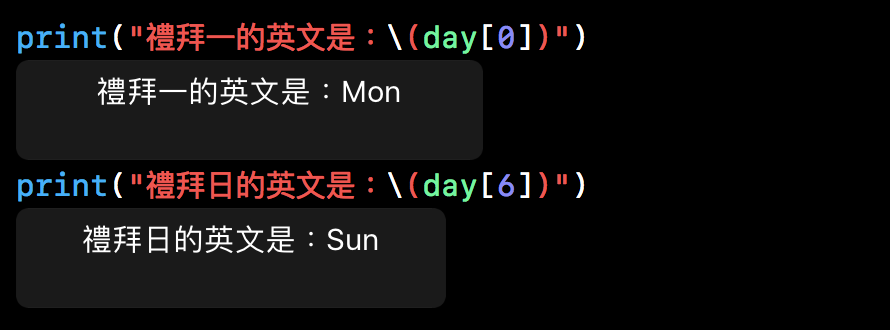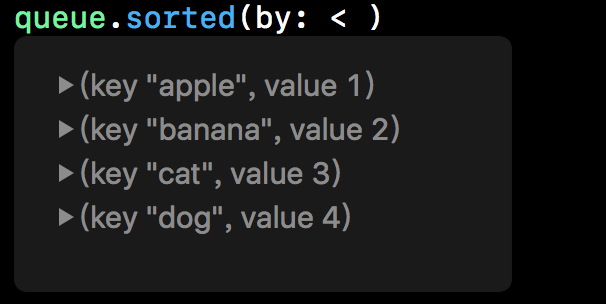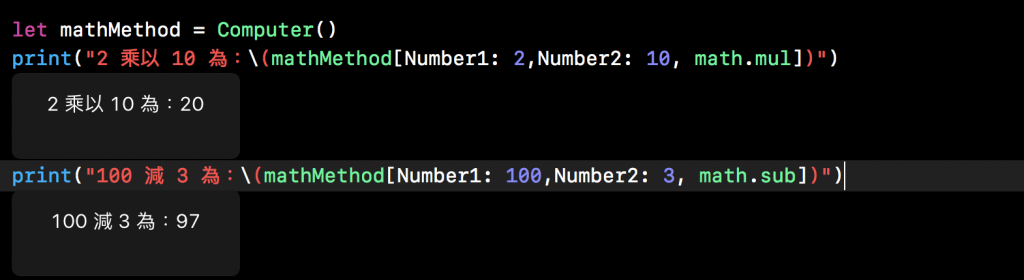#2018 iT 邦幫忙鐵人賽DAY 17
0
Software Development

# 下標 Subscripts

Class、Struct、Enum 可以定義下標，它們均為訪問集合，列表或序列的成員元素的快捷方式。你可以利用下標通過索引值來設置和檢索值，不需要單獨的設置和檢索方法。

# 下標語法

``````subscript(index: Int) -> Int {
get {
// 回傳一個適合的下標值
}
set(newValue) {
// 在這裡執行適合的設置操作
}
}
``````

``````subscript(index: Int) -> Int {
// 回傳一個適合的下標值
}
``````

``````struct Multiplication {
let number:Int
subscript(index:Int) -> Int{
return number * index
}
}

let mul = Multiplication(number: 10)
````````````class Week {
var daysArray = ["Mon","Thu","Wed","Thr","Fri","Sat","Sun"]
subscript(index:Int) -> String {
get {
return daysArray[index]
}
set(newValue){
self.daysArray[index] = newValue
}
}
}
var day = Week()
``````# 下標用法

“下標”的確切含義取決於其使用的上下文。下標通常用作訪問集合，列表或序列中成員元素的快捷方式。你可以自由的用最適合的方式為特定的 class 或 struct 的功能實現下標。

``````var queue = [ "apple":1 , "banana":2 , "cat": 3]
queue["dog"] =  4
//之後我們也可以利用相同的類型 [String : Int]，加入一個 "dog" 鍵值和 Int 值為 4 到 queue 中
``````# 下標選項

``````enum math{
}

class Computer {
var result = 0

func calculate(Number1 a:Int ,Number2 b:Int , method:math) -> Int {
switch method {
result = a + b
case .sub:
result = a - b
case .mul:
result = a * b
case .div:
result = a / b
}
return result
}
// 下標
subscript(Number1 a:Int ,Number2 b:Int , method:math) -> Int {
result  = calculate(Number1: a, Number2: b, method: method)
return result
}
}
``````Swift 菜鳥的30天300